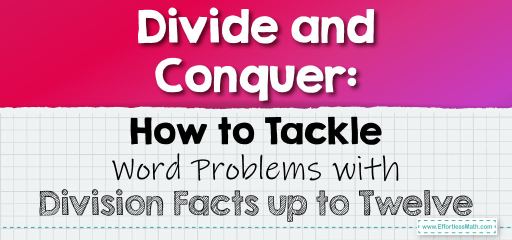# Divide and Conquer: How to Tackle Word Problems with Division Facts up to Twelve

Division is a fundamental operation in mathematics, and mastering division facts up to twelve is crucial for tackling a variety of real-world problems. Let's explore some word problems that involve these division facts.## Division Facts to Twelve in Word Problems

### Example 1:

Liam has $$36$$ cookies. He wants to share them equally among his $$9$$ friends, including himself. How many cookies will each friend get?

Solution Process:

Divide the total number of cookies by the number of friends: $$36 \div 9 = 4$$.

Each friend will get $$4$$ cookies.

The Absolute Best Book for 5th Grade Students

### Example 2:

A school supply store packs pencils in boxes of $$12$$. If they have $$144$$ pencils, how many boxes will they need?

Solution Process:

Divide the total number of pencils by the number in each box: $$144 \div 12 = 12$$.

They will need $$12$$ boxes.

Division word problems often revolve around sharing or grouping items equally. By understanding the division facts up to twelve, you can easily solve these problems and apply this knowledge to various real-world scenarios. Whether it’s sharing resources, distributing items, or grouping entities, division plays a pivotal role. Keep practicing with different word problems to become adept at using division facts in practical situations!

### Practice Questions:

1. A farmer has $$60$$ apples and wants to pack them in bags of $$5$$. How many bags will he need?

2. There are $$84$$ students in a school who need to be divided into teams of $$7$$ for a competition. How many teams will there be?

3. A bakery made $$108$$ muffins and wants to pack them in dozens. How many packs will they make?

4. A library has $$48$$ books and wants to distribute them equally among $$6$$ tables. How many books will each table get?

5. A factory produces $$72$$ toys and packs them in boxes of $$6$$. How many boxes will they use?

A Perfect Book for Grade 5 Math Word Problems!

1. $$12$$ bags

2. $$12$$ teams

3. $$9$$ packs

4. $$8$$ books per table

5. $$12$$ boxes

The Best Math Books for Elementary Students

### What people say about "Divide and Conquer: How to Tackle Word Problems with Division Facts up to Twelve - Effortless Math: We Help Students Learn to LOVE Mathematics"?

No one replied yet.

X
51% OFF

Limited time only!

Save Over 51%

SAVE $15 It was$29.99 now it is \$14.99